Precalculus : Solve Linear Velocity Problems

Example Questions

Example Question #1 : Angular And Linear Velocity

A plane is traveling Northeast. If the eastern component of it's velocity is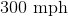, how fast is the plane traveling?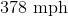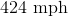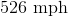Explanation:

Since the plane is traveling NE, we know that it is traveling at an angle of 45 degrees north of east. Therefore, we can represent this in a diagram: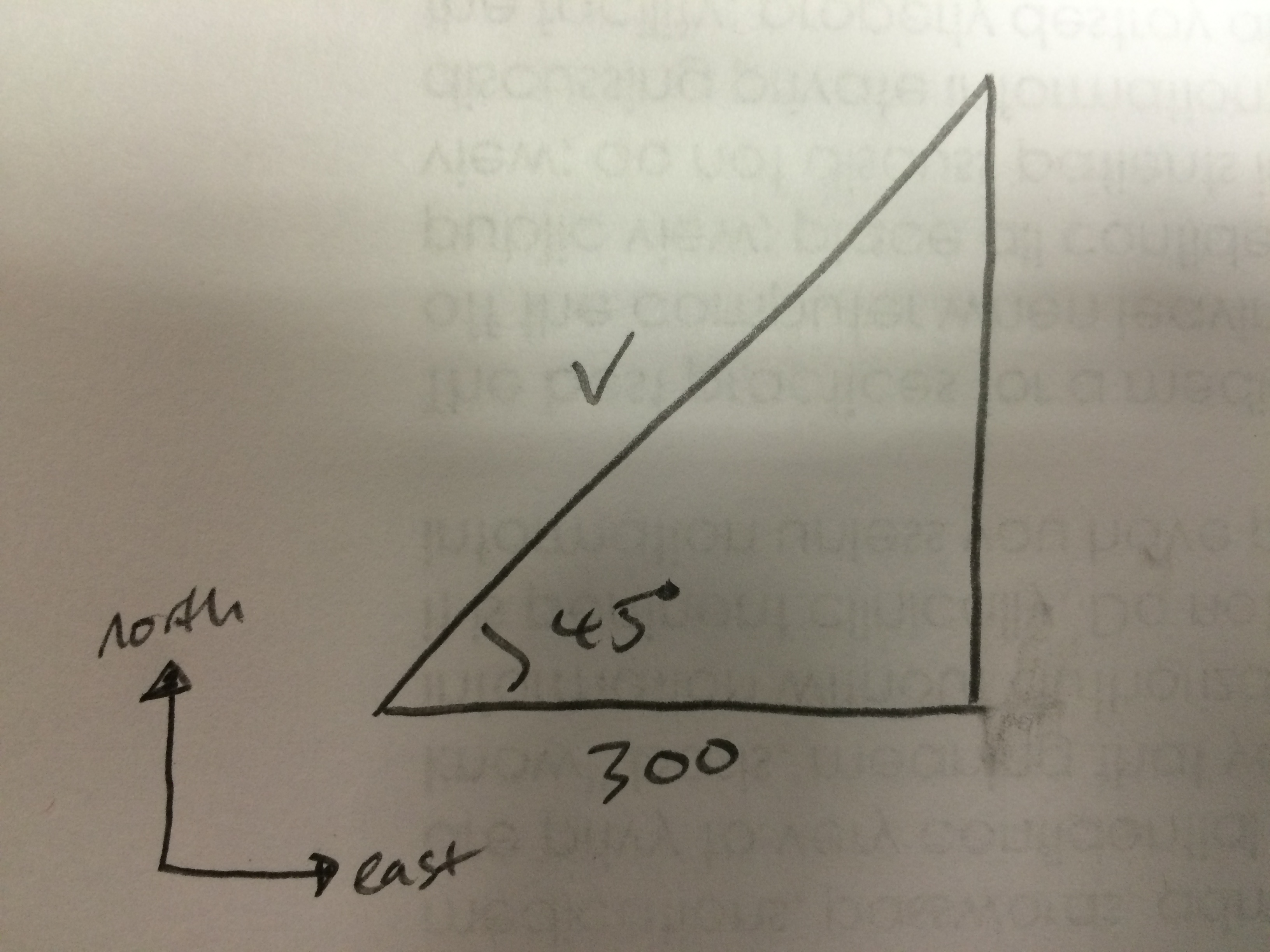We have all of the information we need to solve for the velocity of the plane. We have an angle and a side adjacent to that angle. Therefore, we can use the cosine function to solve for the velocity.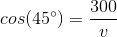Rearranging for v, we get: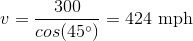Example Question #7 : Angular And Linear Velocity

A car is traveling NW at a speed ofmph. What are the magnitudes of each of its North and West components?

North:mph

West:mph

North:mph

West:mph

North:mph

West:mph

North:mph

West:mph

North:mph

West:mph

North:mph

West:mph

Explanation:

If the car is traveling NW, it means that the angle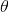isdegrees above the x-axis. Using this angle, and the fact that the magnitude of the velocity ismph, we can find the x and y components, or the west and north components using trigonometry.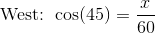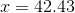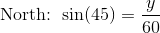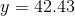Example Question #1 : Angular And Linear Velocity

An object is moving in a circular path. How will its linear velocity change if the diameter of the circular path was decreased by one half?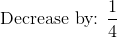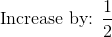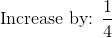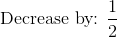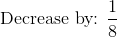Explanation:

Write the formula for the linear velocity.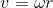The diameter is twice the radius.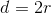If the diameter was decreased by one half, the radius will also decrease by one half.

Therefore: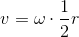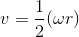The linear velocity will decrease by one half.

Example Question #9 : Angular And Linear Velocity

Find the linear velocity in meters per second of an object if it tookhours to travel a distance ofkilometers. Round to the nearest integer.Explanation:

Write the linear velocity formula.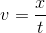Since the answer is required in meters per second, two conversions will need to be made.

Convert 30 kilometers to meters.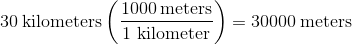Convert 2 hours to seconds.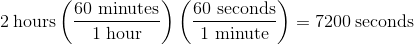Apply the formula.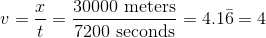Example Question #10 : Angular And Linear Velocity

The second hand of a clock is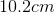long. Find the linear speed of the end of this second hand.Explanation:

Linear velocity is defined as distance over a period of time. For instance if a person ran 1 mile or approximately 1600 meters in 7 minutes, the they would have covered about 230 meters per minute. Let's assume this person was running around a track. We could also measure their speed from a central angle and represent their speed as the amount of degrees (or radians) they ran around per unit time as well. This is considered angular speed. A perfect example of both are the hands on a clock. There is a relationship between arc length which we designate s, the radius r, and the angle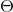(theta). The relationship is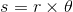. So the length of the arc (s) is equal to the radius of the circle the arc is on and what section of the pie it covers ( this is akin to how many degrees our track runner ran "through" or around). Thus, if we wanted the linear speed around a circle we could say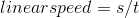or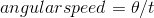. Key measurements that you will need to know are how many degrees in a circle of which there are 360 or. Before you follow the step by step solution below, go back and see if you can use this new information to arrive at the correct answer.

The actual answer is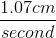.

To see why, note that the second hand spins around a total of 360 degrees or.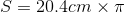.

And how long does it take for the hand to go around? Linear speed of the clock second hand is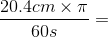(rounded answer).

Example Question #1 : Solve Linear Velocity Problems

A clock has a second hand measuring 12cm. What is the linear speed of the tip of the second hand?None of these/Explanation:

Linear speed is equal to the arc length traversed divided by the time. We use s to denote arc length.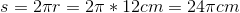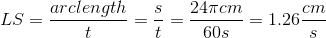All Precalculus Resources